## 竞价系统理论

1. 该问题要解决的是将广告对象 $a = \lbrace 1, 2, … A \rbrace$ 排放到位置 $s = \lbrace 1, 2, …, S \rbrace$，这里的位置主要针对搜索广告而言
2. 假设对象 $a$ 的出价(bid)为 $b_a$ , 而其对位置 $s$ 的计价为 $u_{as} = v_ax_s ,(x_1>x_2 >…>x_S)$， $v_a$ 视为点击价值，$x_s$ 视为点击率，该模型可近似描述广告系统竞价问题(对显示广告，可以认为S = 1，但是与搜索广告的不同点在于搜索广告可以一个都不出，而显示广告至少要出一个)
3. 可以将问题描述成一个对称纳什均衡(Symmetric Nash equilibrium)
其目标是 $(v_s – p_s)x_s >= (v_s – p_t)x_t$, 其中 $p_t = b_{s+1}$,这里的 $p_s$ 表示根据广告的位置实际的收费情况，$b_{s+1}$ 在位置 $s+1$ 的广告的出价；寻找收入最大化且稳定的纳什均衡状态是竞价系统设计的关键

$$p_s = b_{s+1} + 1$$

• 竞价系统(Auction system)
• 淡化广告位概念：卖的是人群，而不是媒体，媒体已经变成了一个载体
• 最合适的计价方式为CPC（Cost Per Click）: 非实时竞价方式，广告网络估计CTR，广告主估计每个广告的价值
• 不足：不易支持定制化用户划分：广告主只能购买广告网络指定的关键词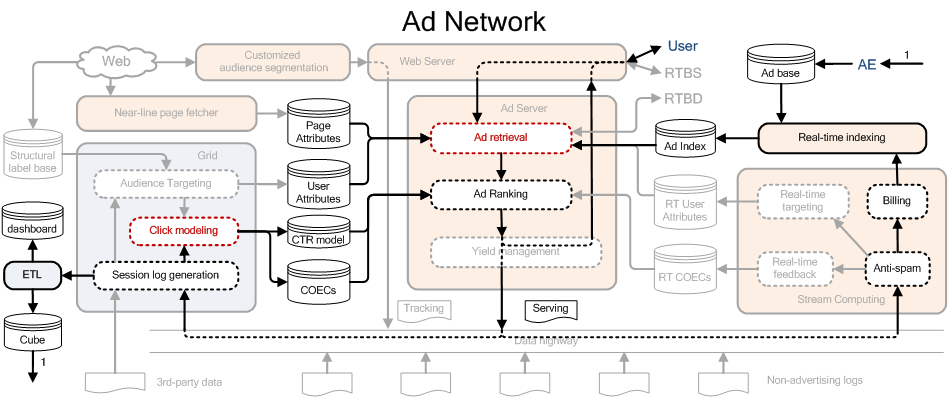1. User 开始浏览某个页面时，Ad retrieval 根据 UserUser Attributes 和页面的 Page AttributesAd Index 中找到相关的广告(如果有广告主将广告新加进来时，也要通过 Real-time indexing 将广告加入 Ad Index 中)
2. 对相关广告根据 eCPM 进行排序，由于点击的 value 已经由广告主决定，这时只需要预估点击率即可。
3. 进行排序后需要将排序的结果及其用户后续的点击行为记录到日志中，从而便于改进点击率预估模型，同时通过流式计算平台进行反作弊监测以及对广告主收费（如果用户有点击行为的话）。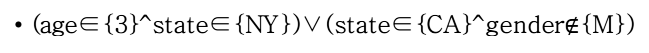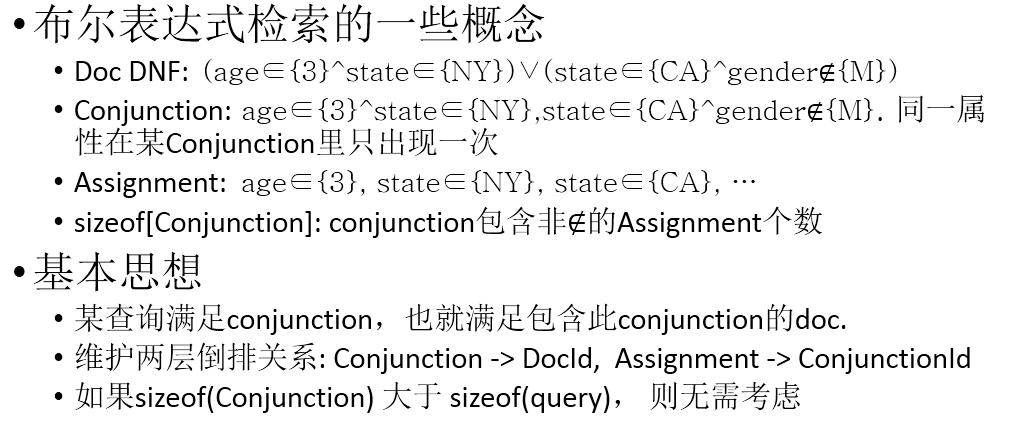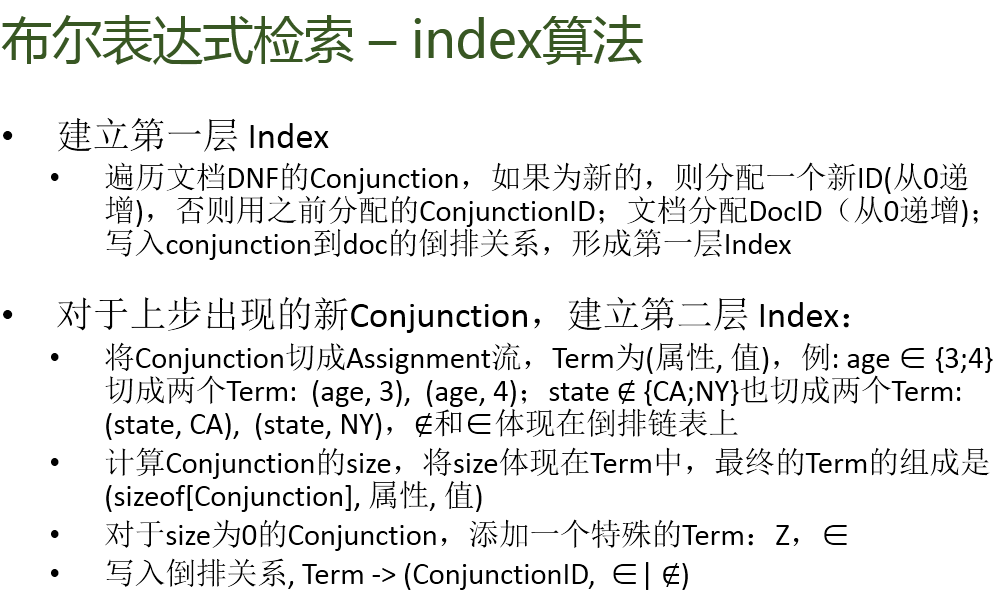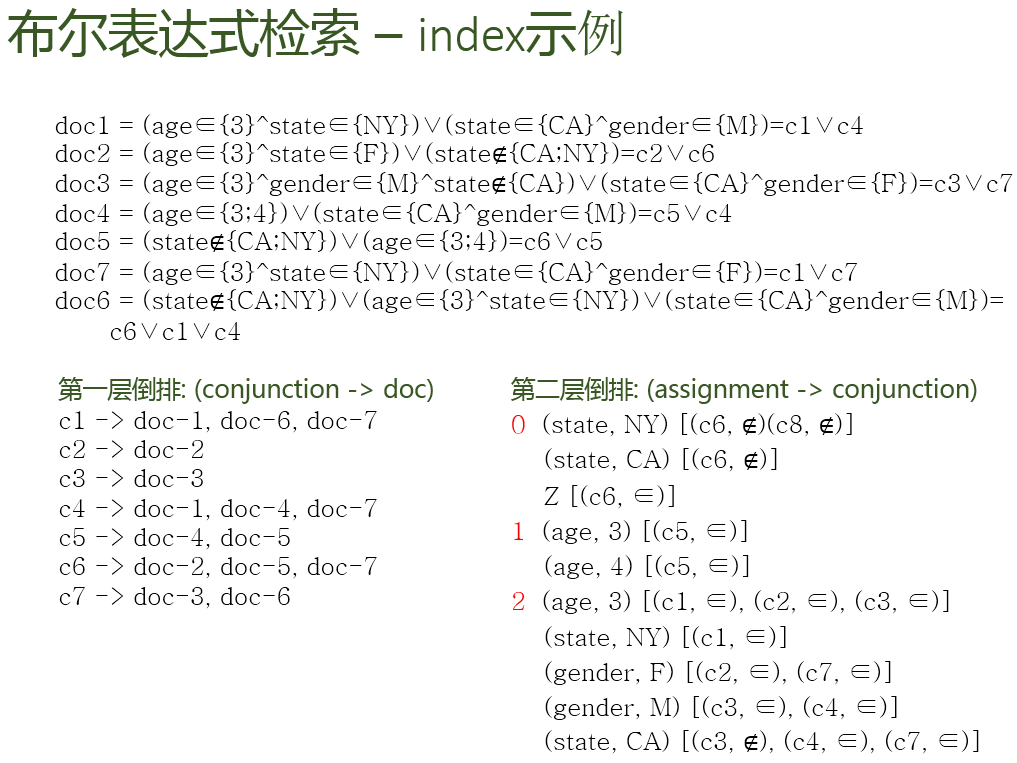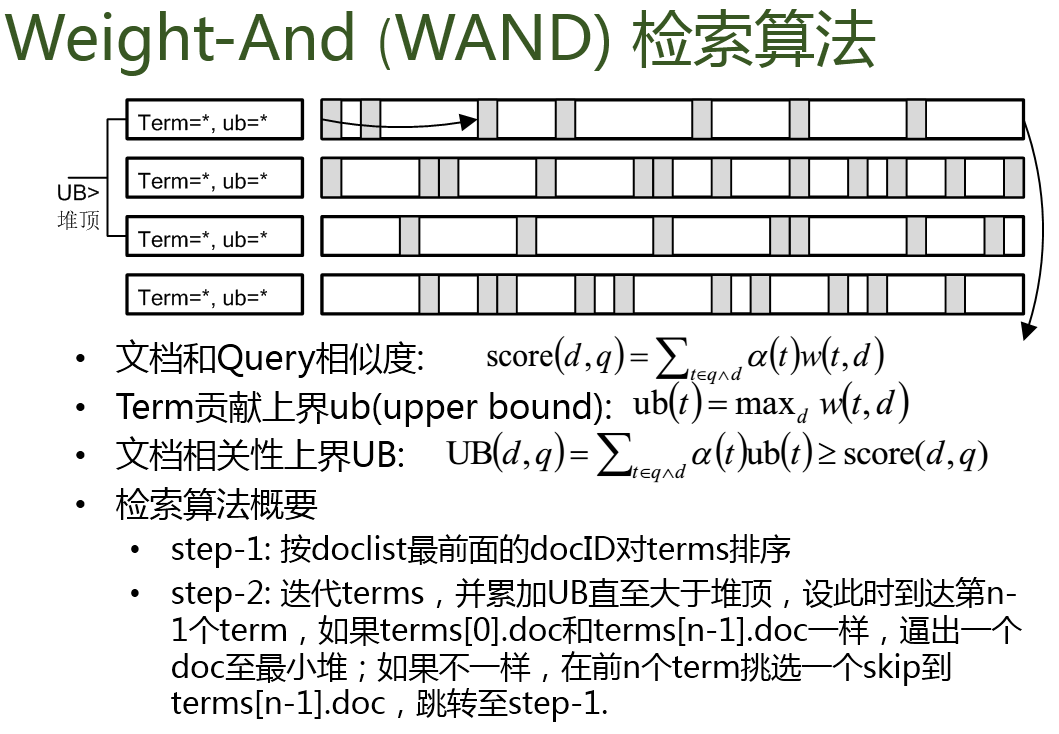## 一致性问题

### Zookeeper

Zookeeper 是在基于消息传递通信模型的分布式环境下解决一致性问题的基础服务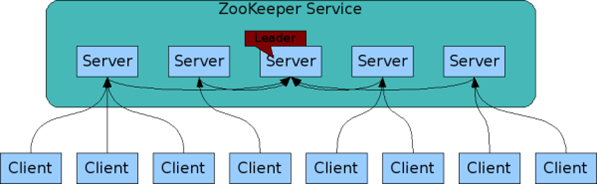### Paxos 算法概念

1. value只有在被提出后才能被批准
2. 在一次算法的执行实例中，只批准一个value
3. learners只能获得被批准的value

1. P选择某提案编号n并将 prepare请求发给A中的某多数派；
2. A收到消息后，若n大于它已经回复的所有消息，则将自己上次接受的提案回复给P，并承诺不再回复小于n的提案；

1. 当P收到了多数A回复后，进入批准阶段。它要向回复请求的 A发送 accept 请求，包括编号 n 和根据约束决定的 value
2. 在不违背向其他P的承诺的前提下，A收到请求后即接受。

## 点击率预测

$$u(a,u,c) = p(click|a,u,c)$$

## 逻辑回归

### 动态特征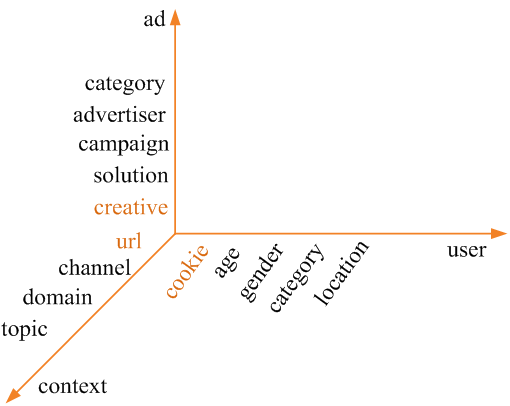1. 工程架构扩展性强，变 features 不变 model(与在线学习相比)
2. 对新 $(a, u, c)$ 组合有较强back-off能力

1. 在线特征的存储量大，更新要求高

• gender(u) and topic(c)
• Category(a) and category(u)
• creative(a)
• gender(u)

### 优化方法

#### L-BFGS

BFGS (Broyden, Fletcher, Goldfarb, and Shanno) 是Quasi-Newton方法的一种， 思路为用函数值和特征的变化量来近似Hession矩阵，以保证正定性，并减少计算量。

BFGS方法Hession计算公式如下 (空间复杂度为 $O(n^2)$ )：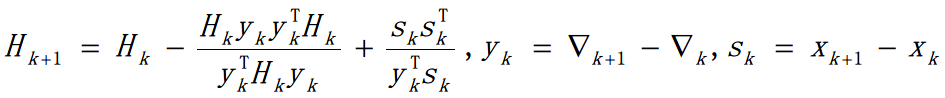L(imited memory)-BFGS 是为了解决 BFGS 的空间复杂度问题。将 nxn 的Hession阵用下图方式加以近似($B_k$ 为Hession近似)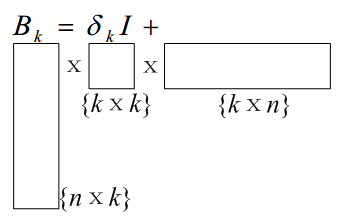Alternating Direction Method of Multipliers 的形式

$$\min f(x)+g(z)\\ s.t. Ax + Bz = c$$

Augmented Lagrangian及迭代解法如下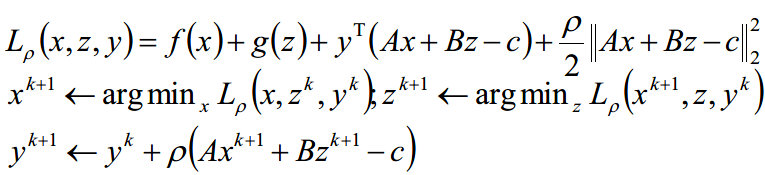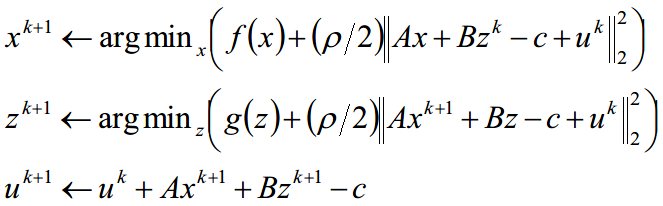$$\min \sum_{i=1}^{N}\sigma (w_ix_i)+r(z)\\ s.t. w - z = 0$$

$$w_i^{k+1} \leftarrow \arg \min_{w_i} (\sigma(w_ix_i) + \frac{\rho}{2}||w_i - z^k + \mu_i^k||_2^2)\\ z^{k+1} \leftarrow \arg \min_{z} (r(z) + \frac{N\rho}{2}||z^k - \overline w^{k+1}+ \overline \mu_k||_2^2)\\ \mu_i^{k+1} \leftarrow \mu_i^k + w_i^{k+1} - z^{k+1}$$

## 探索与利用(Explore and exploit， E&E)

1. 通常描述为 Multi-arm Bandit (MAB) 问题: 有限个 arms(或称收益提供者) $a$, 每个有确定有限的期望收益 $E(r_{t,a})$
2. 在每个时刻t,我们必须从arms中选择一个,最终目标是优化整体收益
基本方法为 $\epsilon$–greedy: 将 $\epsilon$ 比例的小部分流量用于随机探索

1. 海量的组合空间需要被探索
2. 各个arm的期望收益是动态变化的

UCB

1. β-UCB策略: 依一个很大的概率, 我们选择非最优arms的次数存在着一个上界, 该上界与总的选择次数无关
2. UCB-tuned策略: 我们已选择的次数越多, 就越可以自信地抛弃不太有前途(但仍有可能最优)的arm.

Contextual Bandit

• 对每次展示，每个 arm (广告) $a$ 有一个对应的特征矢量 $x(u,a)$
• 用此特征矢量代替 arm 本身进行 Bandit 决策